Click to Chat

1800-1023-196

+91-120-4616500

CART 0

• 0

MY CART (5)

Use Coupon: CART20 and get 20% off on all online Study Material

ITEM
DETAILS
MRP
DISCOUNT
FINAL PRICE
Total Price: Rs.

There are no items in this cart.
Continue Shopping```Ionic Bonding

Table of Content

Electrovalency

Criteria for Ionic Bond Formation

Energy Change During the Formation of Ionic Bond

Determination of Lattice Energy

Characteristics of Ionic Compounds

you can also Refer to

Electrovalency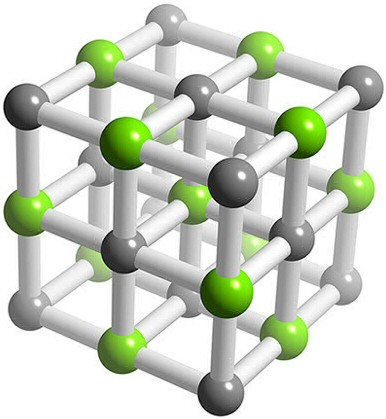This type of valency involves transfer of electrons from one atom to another, whereby each atom may attain octet in their outermost shell. The resulting ions that are formed by gain or loss of electrons are held together by electrostatic force of attraction due to opposite nature of their charges. The reaction between potassium and chlorine to form potassium chloride is an example of this type of valency.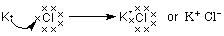Here potassium has one electron excess of it’s octet and chlorine has one deficit of octet. So potassium donates it’s electron to chlorine forming an ionic bond.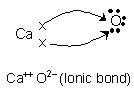Here the oxygen accepts two electrons from calcium atom. It may be noted that ionic bond is not a true bond as there is no proper overlap of orbitals.

Criteria for Ionic Bond Formation

One of the species must have electrons in excess of octet while the other should be deficit of octet. Does this mean that all substance having surplus electron and species having deficient electron would form ionic bond? The answer is obviously no. Now you should ask why? The reasoning is that in an ionic bond one of the species is cation and the other is anion. To form a cation from a neutral atom energy must be supplied to remove the electron and that energy is called ionization energy. Now it is obvious that lower the ionization energy of the element the easier it is to remove the electron. To form the anion, an electron adds up to a neutral atom and in this process energy is released. This process is called electron affinity.

So for an ionic bond one of the species must have low ionization energy and the other should have high electron affinity. Low ionization energy is mainly exhibited by the alkali and alkaline earth metals and high electron affinity by the halogen and chalcogens. Therefore this group of elements are predominant in the field of ionic bonding.

Energy Change During the Formation of Ionic Bond

The formation of ionic bond can be consider to proceed in three steps

(a) Formation of gaseous cations
A(g) + I.E. → A+ (g) + e–

The energy required for this step is called ionization energy (I.E)

(b) Formation of gaseous anions

X(g) + e– → X–(g) + E.A

The energy released from this step is called electron affinity (E.A.)

(c) Packing of ions of opposite charges to form ionic solid

A+(g) + X–(g) → AX(s) + energy

The energy released in this step is called lattice energy.

Now for stable ionic bonding the total energy released should be more than the energy required.

From the above discussion we can develop the factors which favour formation of ionic bond and also determine its strength. These factors have been discussed below:

(a) Ionization energy: In the formation of ionic bond a metal atom loses electron to form cation. This process required energy equal to the ionization energy. Lesser the value of ionization energy, greater is the tendency of the atom to form cation. For example, alkali metals form cations quite easily because of the low values of ionization energies.

(b) Electron affinity: Electron affinity is the energy released when gaseous atom accepts electron to form a negative ion. Thus, the value of electron affinity gives the tendency of an atom to form anion. Now greater the value of electron affinity more is the tendency of an atom to form anion. For example, halogens having highest electron affinities within their respective periods to form ionic compounds with metals very easily.

(c) Lattice energy: Once the gaseous ions are formed, the ions of opposite charges come close together and pack up three dimensionally in a definite geometric pattern to form ionic crystal.

Since the packing of ions of opposite charges takes place as a result of attractive force between them, the process is accompanied with the release of energy referred to as lattice energy. Lattice energy may be defined as the amount of energy released when one mole of ionic solid is formed by the close packing of gaseous ion.

In short, the conditions for the stable ionic bonding are:

(a) I.E. of cation forming atom should be low:

(b) E.A. of anion – forming atom should be high;

(c) Lattice energy should be high.

Determination of Lattice Energy

The direct calculation of lattice enthalpy is quite difficult because the required data is often not available. Therefore lattice enthalpy is determined indirectly by the use of the Born – Haber cycle. The cycle uses ionization enthalpies, electron gain enthalpies and other data for the calculation of lattice enthalpies. The procedure is based on the Hess’s law, which states that the enthalpy of a reaction is the same, whether it takes place in a single step or in more than one step. In order to understand it let us consider the energy changes during the formation of sodium chloride from metallic sodium and chlorine gas. The net energy change during the process is represented by ΔHf.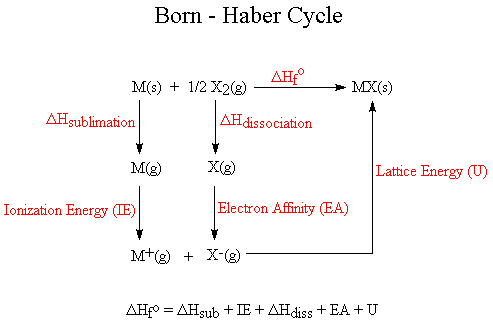For example:  Born- Haber Cycle for Soidum ChlorideRefer to the following video for lattice energy and Born- Haber Cycle

Solved Problem on Born- Haber Cycle

Calculate the lattice enthalpy of MgBr2. Given that

Enthalpy of formation of MgBr2 = -524 kJ mol–1

Some of first & second ionization enthalpy (IE1 + IE2 ) = 148 kJ mol-1

Sublimation energy of Mg = +2187 kJ mol–1

Vaporization energy of Br2(I) = +31kJ mol–1

Dissociation energy of Br2(g) = +193kJ mol–1

Electron gain enthalpy of Br(g) = -331 kJ mol–1

Solution: ΔHof = S + I.E. + ΔHvap + D + 2 × E.A. + U

Or  U = ΔHof – (S + I.E. + ΔHvap + D + 2 × E.A.)

Or U = -524 - [2187 - 148 + 31 + 193 + 2 × (-331)]

= – 524 – 1897 = –2421 kJ mol–1

Characteristics of Ionic Compounds

The following are some of the general properties shown by these compounds

(i) Crystalline nature :  These compounds are usually crystalline in nature with constituent units as ions. Force of attraction between the ions is non-directional and extends in all directions. Each ion is surrounded by a number of oppositely charged ions and this number is called co-ordination number. Hence they form three dimensional solid aggregates. Since electrostatic forces of attraction act in all directions, therefore, the ionic compounds do not posses directional characteristic and hence do not show stereoisomerism.

(ii) Due to strong electrostatic attraction between these ions, the ionic compounds have high melting and boiling points.

(iii) In solid state the ions are strongly attracted and hence are not free to move. Therefore, in solid state, ionic compounds do not conduct electricity. However, in fused state or in aqueous solution, the ions are free to move and hence conduct electricity.

(iv) Solubility : Ionic compounds are fairly soluble in polar solvents and insoluble in non-polar solvents. This is because the polar solvents have high values of dielectric constant which defined as the capacity of the solvent to weaken the force of attraction between the electrical charges immersed in that solvent. This is why water, having high value of dielectric constant, is one of the best solvents.

The solubility in polar solvents like water can also be explained by the dipole nature of water where the oxygen of water is the negative and hydrogen being positive, water molecules pull the ions of the ionic compound from the crystal lattice. These ions are then surrounded by water dipoles with the oppositely charged ends directed towards them. These solvated ions lead an independent existence and are thus dissolved in water. The electrovalent compound dissolves in the solvent if the value of the salvation energy is higher than the   lattice energy of that compounds.

AB + Lattice energy → A+ + B–

These ions are surrounded by solvent molecules. This process is exothermic and is called salvation.

A+ + x(solv.) → [A(solv.)x]+ + energy

B– + y(solv.) → [B(solv.)y]– + energy

The value of solvation energy depend on the relative size of the ions. Smaller the ions more is the solvation. The non-polar solvents do not solvate ions and thus do not release energy due to which they do not dissolve ionic compounds.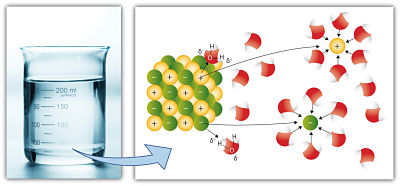(v) Ionic reactions: Ionic compound furnish ions in solutions. Chemical reactions are due to the presence of these ions. For example

Na2SO4 → 2Na+ + SO42–

BaCl2 → Ba2+ + 2Cl–

you can also Refer to

JEE  Chemistry Syllabus

Reference books of Physical Chemistry

To read more, Buy study materials of Chemical Bonding comprising study notes, revision notes, video lectures, previous year solved questions etc. Also browse for more study materials on Chemistry here
```### Course Features

• 731 Video Lectures
• Revision Notes
• Previous Year Papers
• Mind Map
• Study Planner
• NCERT Solutions
• Discussion Forum
• Test paper with Video Solution